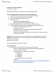# BIO120H1 Lecture Notes - Lecture 5: Exponential Growth, Population Ecology, Inflection Point

42 views3 pagesyifanyang and 39659 others unlocked36
Verified Note
36 documents

## Document Summary

Lecture 5: population ecology: models without age structure. An approach to population modeling assuming that time flows continuously and that change can occur at every instant. Differential equations are used, time steps are infinitely small: use concept of limits &calculus; growth is smooth. An approach to population modeling that uses discrete time intervals, generally corresponding to intervals between reproductive periods. Difference equations are used, time steps are discrete units (days, years, etc. use iterated recursion equations; growth is stepwise and bumpy. Per capita: the rate of growth on a per-individual basis. Geometric growth: increase/decrease in a population as measured over discrete intervals in which the increment is proportional to the number of individuals at the beginning of the interval. When we combine death, birth, emigrant and immigrant, we will have a ratio (factor by which population changes over one time unit net reproductive rate). Discrete time can be expressed as geometric growth: